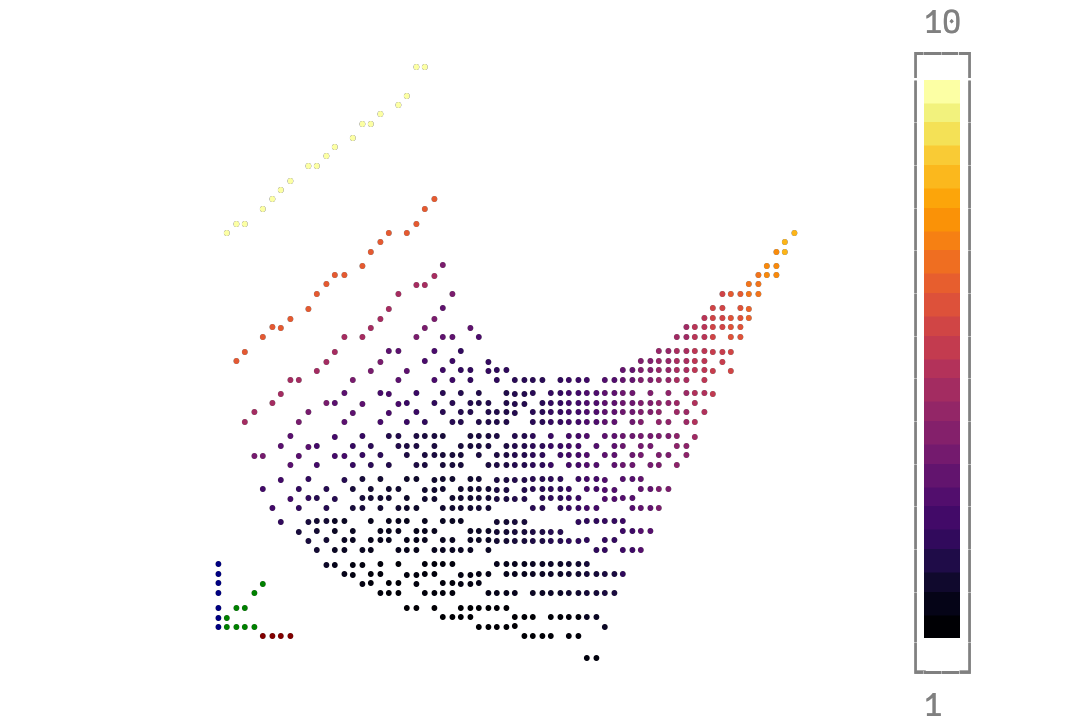# 3D surface with axis guidesusing Plots
unicodeplots()
xs = collect(0.1:0.05:2.0)
ys = collect(0.2:0.1:2.0)
X = [x for x = xs for _ = ys]
Y = [y for _ = xs for y = ys]
Z = ((x, y)->begin
1 / x + y * x ^ 2
end)
surface(X, Y, Z.(X, Y), xlabel = "longer xlabel", ylabel = "longer ylabel", zlabel = "longer zlabel")